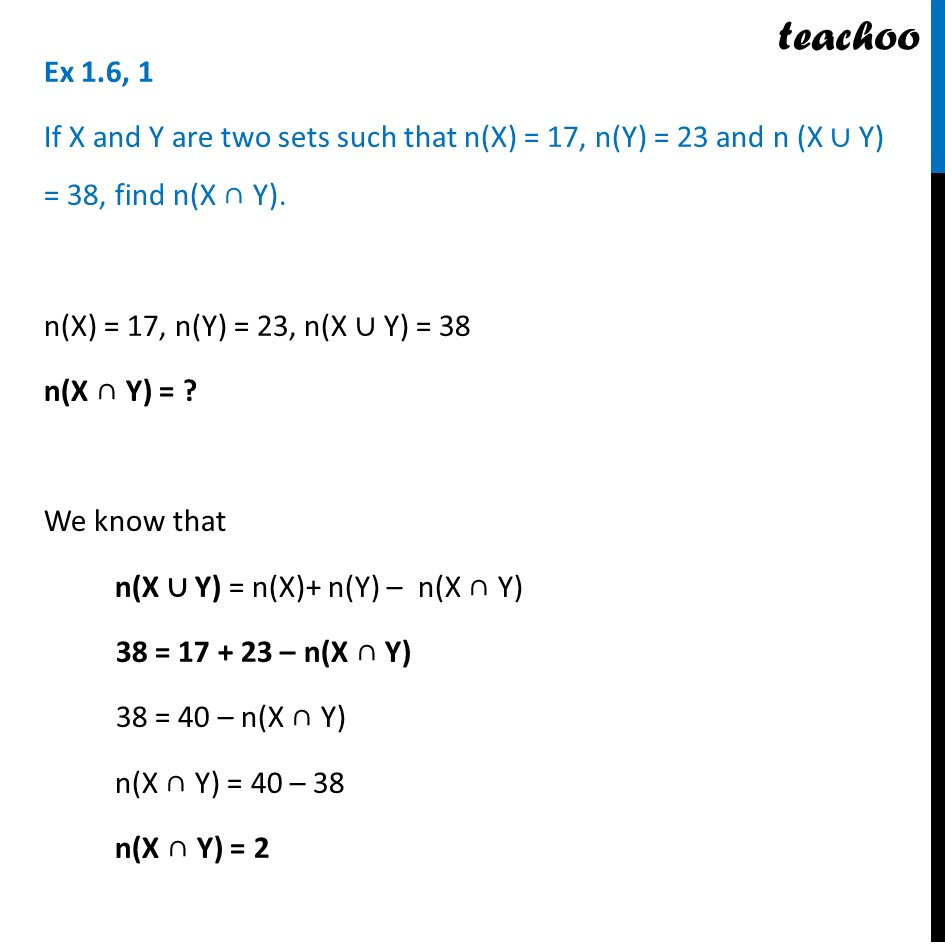Number of elements in Set Formula

Chapter 1 Class 11 Sets
Serial order wiseLearn in your speed, with individual attention - Teachoo Maths 1-on-1 Class

### Transcript

Question 1 If X and Y are two sets such that n(X) = 17, n(Y) = 23 and n (X ∪ Y) = 38, find n(X ∩ Y). n(X) = 17, n(Y) = 23, n(X ∪ Y) = 38 n(X ∩ Y) = ? We know that n(X ∪ Y) = n(X)+ n(Y) – n(X ∩ Y) 38 = 17 + 23 – n(X ∩ Y) 38 = 40 – n(X ∩ Y) n(X ∩ Y) = 40 – 38 n(X ∩ Y) = 2